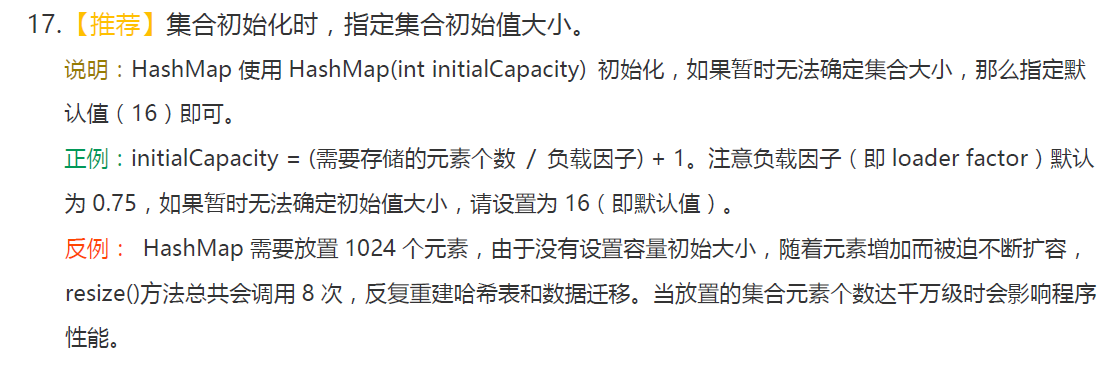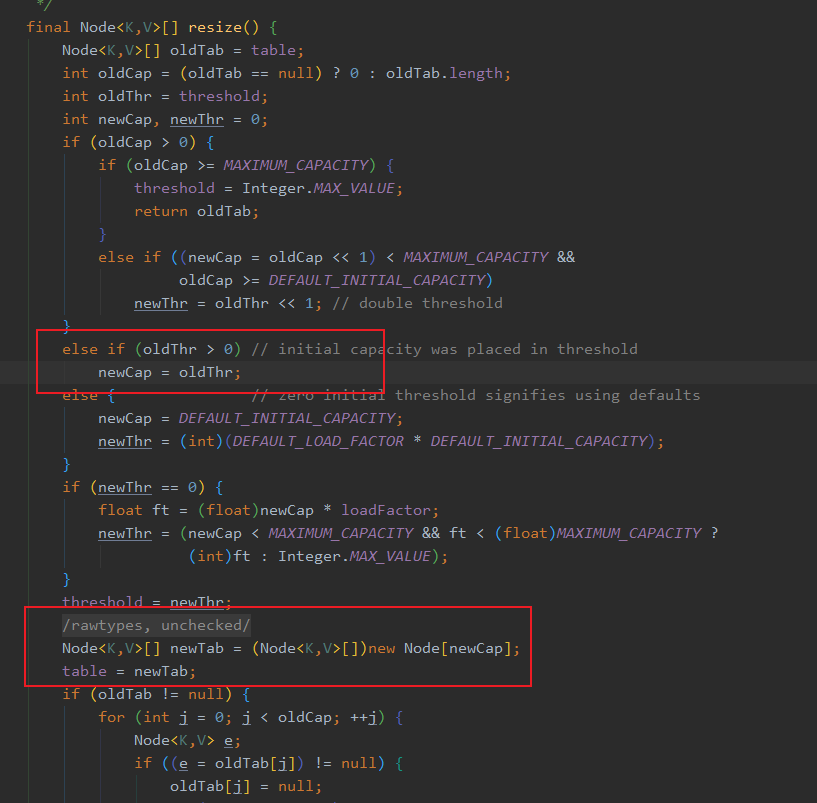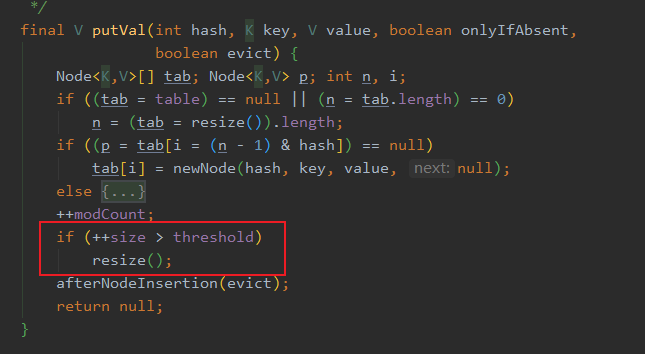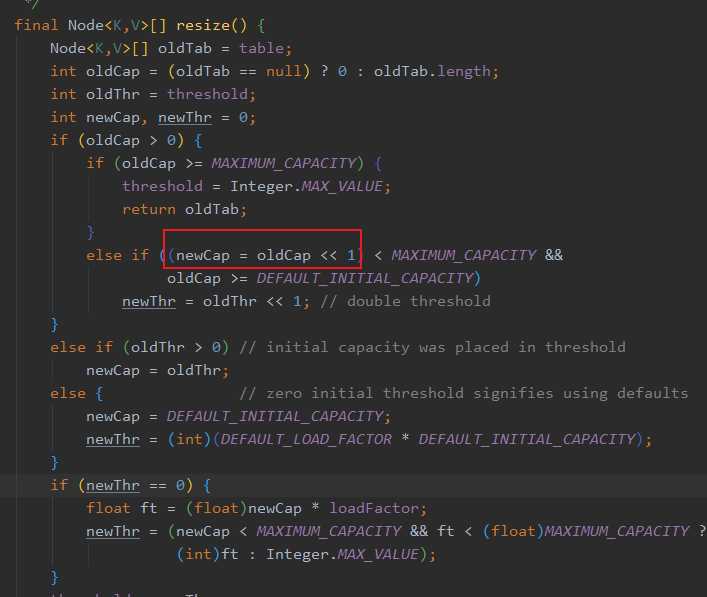# 如何设置 HashMap 初始化大小Hex## 说明

### HashMap 中几个属性值说明

capacity:容量，Node 数组的大小（桶的数量），值为 2 的 N 次幂

threshold:扩容阈值，等于 capacity * load factor 当 size 大于此值时进行扩容

loadFactor：负载因子，默认为 0.75f

#### capacity 与 initialCapacity 的关系

HashMap设置初始容器的构造方法如下，也就是程序员可以指定initialCapacity

  public HashMap(int initialCapacity) {.        // DEFAULT_LOAD_FACTOR = 0.75f        this(initialCapacity, DEFAULT_LOAD_FACTOR);    }    public HashMap(int initialCapacity, float loadFactor) {        if (initialCapacity < 0)            throw new IllegalArgumentException("Illegal initial capacity: " +                                               initialCapacity);        if (initialCapacity > MAXIMUM_CAPACITY)            initialCapacity = MAXIMUM_CAPACITY;        if (loadFactor <= 0 || Float.isNaN(loadFactor))            throw new IllegalArgumentException("Illegal load factor: " +                                               loadFactor);        this.loadFactor = loadFactor;        this.threshold = tableSizeFor(initialCapacity);    }

  /**     * Returns a power of two size for the given target capacity.     */    static final int tableSizeFor(int cap) {        int n = cap - 1;        n |= n >>> 1;        n |= n >>> 2;        n |= n >>> 4;        n |= n >>> 8;        n |= n >>> 16;        return (n < 0) ? 1 : (n >= MAXIMUM_CAPACITY) ? MAXIMUM_CAPACITY : n + 1;    }

tableSizeFor 方法最终返回是，比给定 cap 值大且最接近的 2 的幂次方的整数，比如 cap=3，tableSizeFor(3)=4;cap=4，tableSizeFor(4)=4;cap=7，tableSizeFor(7)=8

>>>无符号右移，右移时不关注符号位，右移后前面补 0

  final int capacity() {        return (table != null) ? table.length :            (threshold > 0) ? threshold :            DEFAULT_INITIAL_CAPACITY;    }

new HashMap(int initialCapacity)初始化HashMap时，还未 put 元素时，table==null，那么 capacity 的值就等于上面计算出的threshold，即capacity的值为比initialCapacity大且最接近的 2 的幂次方的整数。

• 1.取大于（需要存储的元素数量）且最接近的 2 的幂次方的整数，即为 C1，比如要存储 3 个元素，则 C1=4；

• 2.计算 C1 * loadFactor，记为 L1，比如要存储 3 个元素，则 L1=3；

• 3.比较 L1 与（需要存储的元素数量），如果 L1<（需要存储的元素数量）,说明会触发扩容，则 initialCapacity 取大于 C1 且最接近 C1 的 2 的幂次方的整数；如果 L1>=（需要存储的元素数量）,说明不会触发扩容，则 initialCapacity 取 C1。

### 扩容

  public V put(K key, V value) {        return putVal(hash(key), key, value, false, true);    }    /**     * Implements Map.put and related methods.     *     * @param hash hash for key     * @param key the key     * @param value the value to put     * @param onlyIfAbsent if true, don't change existing value     * @param evict if false, the table is in creation mode.     * @return previous value, or null if none     */    final V putVal(int hash, K key, V value, boolean onlyIfAbsent,                   boolean evict) {        Node<K,V>[] tab; Node<K,V> p; int n, i;        // 第一次put时table == null,会调用resize()        if ((tab = table) == null || (n = tab.length) == 0)            n = (tab = resize()).length;        if ((p = tab[i = (n - 1) & hash]) == null)            tab[i] = newNode(hash, key, value, null);        else {            Node<K,V> e; K k;            if (p.hash == hash &&                ((k = p.key) == key || (key != null && key.equals(k))))                e = p;            else if (p instanceof TreeNode)                e = ((TreeNode<K,V>)p).putTreeVal(this, tab, hash, key, value);            else {                for (int binCount = 0; ; ++binCount) {                    if ((e = p.next) == null) {                        p.next = newNode(hash, key, value, null);                        if (binCount >= TREEIFY_THRESHOLD - 1) // -1 for 1st                            treeifyBin(tab, hash);                        break;                    }                    if (e.hash == hash &&                        ((k = e.key) == key || (key != null && key.equals(k))))                        break;                    p = e;                }            }            if (e != null) { // existing mapping for key                V oldValue = e.value;                if (!onlyIfAbsent || oldValue == null)                    e.value = value;                afterNodeAccess(e);                return oldValue;            }        }        ++modCount;        if (++size > threshold)            resize();        afterNodeInsertion(evict);        return null;    }

#### 初始化 table

if ((tab = table) == null || (n = tab.length) == 0)            n = (tab = resize()).length;if (newThr == 0) {            // 计算新的阈值            float ft = (float)newCap * loadFactor;            newThr = (newCap < MAXIMUM_CAPACITY && ft < (float)MAXIMUM_CAPACITY ?                      (int)ft : Integer.MAX_VALUE);        }        threshold = newThr;

public class MapDemo {    public static void main(String[] args) throws Exception {        HashMap<String, String> map = new HashMap<>(4);        System.out.println("初始化HashMap后，threshold:" + getThreshold(map));        map.put("book1", "java");        System.out.println("第一次put后，threshold:" + getThreshold(map));    }    /**     * 通过反射获取HashMap的threshold值     * @param map     * @return     * @throws Exception     */    private static int getThreshold(HashMap map) throws Exception {        Field field = map.getClass().getDeclaredField("threshold");        field.setAccessible(true);        int threshold = field.getInt(map);        return threshold;    }}

#### 扩容## 总结

ps 建议 initialCapacity 尽量取 2 的幂次方，虽然不取 2 的幂次方效果也一致,initialCapacity=5 和 initialCapacity=8 的效果是一样的，但建议使用 initialCapacity=8。## 评论